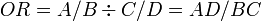# Attributable and Relative Risks, Odds Ratio

## Overview

The term measures of effect includes ratios, differences, relative risks (RR), odds ratios.

Population attributable risk and population attributable risk factor are also measures of effect but more specifically are measures of population impact.

## Ratios and Differences

Risks (defined as the probability of a new occurrence disease among individuals in an initially disease free population, over a defined period of time) can be compared by two ways, either ratios (relative comparison) or difference (absolute comparison).

## Relative Risk (RR)

For a given disease, we can know the risk among exposed (r1), and the risk among unexposed (r0). Hence we can calculate the risk ratio as follows:

RR = r1 / r0

Similarly we can also calculate rate ratios if we know the rate of disease (rate of occurrence of new disease) in an exposed group (r1), and the rate of disease in an unexposed group (r0).

RR = r1 / r0

We can then also stratify the extent of exposure, and calculate rate ratio for each strata. This allows us to compare measure of disease frequency in each level against the baseline frequency (unexposed) level. See the example below:

Death rates from CHD in smokers and non-smokers by age

Age Smokers Rate Non-Smokers Rate Rate Ratio
35-44 0.61 0.11 5.5
45-54 2.40 1.12 2.1
55-64 7.20 4.90 1.5
65-74 14.69 10.83 1.4
75-84 19.18 21.20 0.9
85+ 35.93 32.66 1.1
All Ages 4.29 3.30 1.3

We can make three main remarks about the data:

• the rate increases with age
• smokers have a higher rate than non-smokers
• effect of smoking on the rate of CHD is greater in younger ages

## Odds Ratio (OR)

This is defined as the ratio of odds of disease among exposed (odds1) and odds of disease among unexposed (odds0).

OR = (odds1) / (odds0)
Disease
Yes No Total
Unexposed A B A+B
Exposed C D C+D
Total A+C B+D N = A+B+C+D

Using the above table, we can calculate the odds ratio using this formula:$OR = A/B \div C/D = AD/BC$

Risk difference percentage (RD%) represents the proportion os cases in the exposed grip that were actually caused by the exposure. This is equal to attributable fraction for exposed (see below). It is calculate as follows:

RD% = (r1 − r0) / r1

## Measures of Population Impact

Population attributable risk (PAR) represents the absolute difference between risk (or rate) in the whole population and the risk (or rate) in the unexposed group). It is calculated as follows:

PAR = r − r0

Or, if we already know the rates among exposed (r1), unexposed (r0) as well as the proportion of the population that is exposed (p), we can proceed like this:

PAR = p (r1 − r0)

Population attributable risk fraction (PAF) is the measure of the proportion of all cases in the given population that may be accounted for by the exposure. It can also be caused the "aetiological fraction". If r is the rate in the total population, then we can proceed as follows:

PAF = PAR / r
PAR = r − r0
PAF = (r − r0) / r

PAF can also be calculated if only some limited information is known. If only the rate ratio (RR) and proportion of exposed in the population (p), PAF can be found as follows:

PAF = p (RR − 1) / {p(RR-1) +1 }

Measures of population impact is mostly used for planning public health measures. For example this can be to predict the impact of a change in the distribution of various risk factors on the frequency or incidence of disease in a given population.